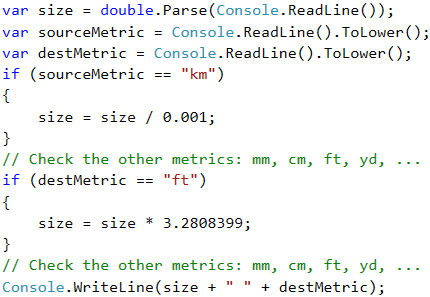Problem: Metric Converter

Write a program that convert a distance between the following 8 units of measure: m, mm, cm, mi, in, km, ft, yd. Use the below table:

Input measure Output measure
1 meter (m) 1000 millimeters (mm)
1 meter (m) 100 centimeters (cm)
1 meter (m) 0.000621371192 miles (mi)
1 meter (m) 39.3700787 inches (in)
1 meter (m) 0.001 kilometers (km)
1 meter (m) 3.2808399 feet (ft)
1 meter (m) 1.0936133 yards (yd)

You have three input lines:

• First line: number for converting.
• Second line: input unit.
• Third line: output unit (for result).

Input Output
12
km
ft
39370.0788
150
mi
in
9503999.99393599
450
yd
km
0.41147999937455

Video: Metric Converter

Watch the video lesson to learn how to solve the "Metric Converter" problem: https://youtu.be/Bd6NgaHhrko.

Hints and Guidelines

We read the input data, and we can add ToLower() function when we read the measuring units. The function will make all letters small. As we can see from the table in the condition, we can only do converting between meters and some other measuring unit. Then, first we have to calculate the number for converting in meters. That's why, we need to make a set of checks to define the input unit and then the output unit.